Function Repository Resource:

# TridiagonalCompanionMatrix

Generate the tridiagonal companion matrix of a univariate polynomial

Contributed by: Jan Mangaldan
 ResourceFunction["TridiagonalCompanionMatrix"][poly,x] gives a tridiagonal companion matrix of poly, treated as a polynomial in x.

## Details

ResourceFunction["TridiagonalCompanionMatrix"] produces a tridiagonal matrix whose characteristic polynomial is a scalar multiple of the original polynomial.
ResourceFunction["TridiagonalCompanionMatrix"] returns a SparseArray object.

## Examples

### Basic Examples (2)

Generate a tridiagonal companion matrix:

 In:=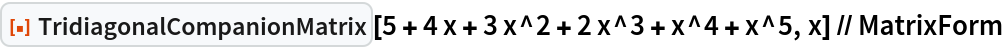Out=The characteristic polynomial of this matrix is a constant multiple of the original polynomial:

 In:=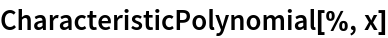Out=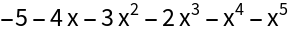### Scope (2)

Tridiagonal companion matrix of a polynomial with complex coefficients:

 In:=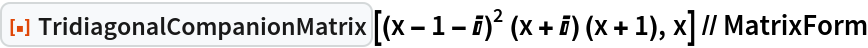Out=Tridiagonal companion matrix of a polynomial with symbolic coefficients:

 In:=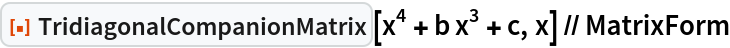Out=### Applications (2)

Numerically find the roots of a polynomial by computing the eigenvalues of its tridiagonal companion matrix:

 In:=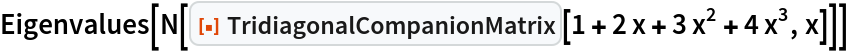Out=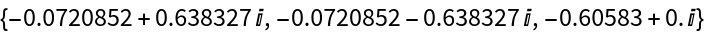Compare with the result of NSolve:

 In:=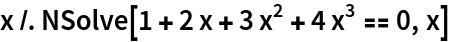Out=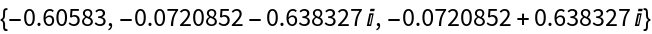### Properties and Relations (4)

A polynomial with real coefficients whose roots are all real has a symmetric tridiagonal companion matrix:

 In:=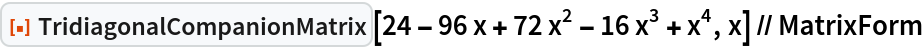Out=In:=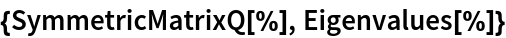Out=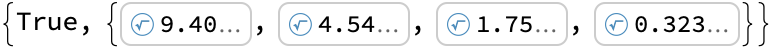If the polynomial has repeated roots, then the companion matrix is symmetric and block diagonal:

 In:=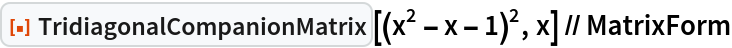Out=In:=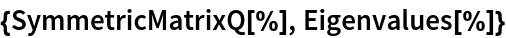Out=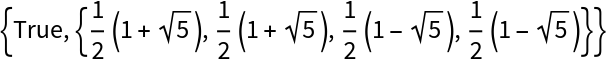A polynomial with real coefficients and complex roots will have a tridiagonal companion matrix with negative entries in its superdiagonal:

 In:=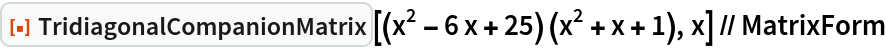Out=In:=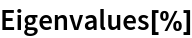Out=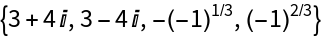If the polynomial has repeated roots, the superdiagonal will also have zero entries:

 In:=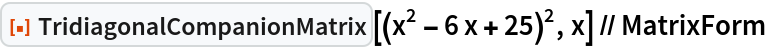Out=In:=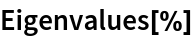Out=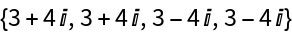## Version History

• 1.0.0 – 04 February 2021